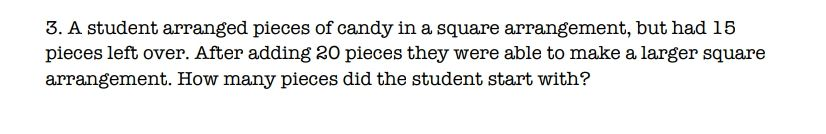### ¿Todavía tienes preguntas de matemáticas?

Pregunte a nuestros tutores expertos
Algebra
Pregunta3. A student arranged pieces of candy in a square arrangement, but had $$15$$ pieces left over. After adding $$20$$ pieces they were able to make a larger square arrangement. How many pieces did the student start with?

$$a^{2} + 15+ 20= b^{2}$$

$$(b+ a)(b- a)= 5\times 7$$

b+a=7

b-a=5

b=6,a=1

so the student starts with 1+15=16 pieces.

Solución
View full explanation on CameraMath App.